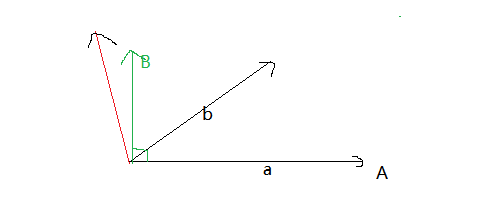MIT 18.06 linear algebra 第十七讲笔记

• Orthogonal basis ${q}_{1},{q}_{2}....{q}_{3}$$q_1,q_2....q_3$
• Orthogonal matrix $Q$$Q$
• Gram-Schmidt $A\to Q$$A\rightarrow Q$
• Orthogonal normal vector

$\left(Q{Q}^{T}\right)\left(Q{Q}^{T}\right)=Q\left({Q}^{T}Q\right){Q}^{T}=QI{Q}^{T}=Q{Q}^{T}$$(QQ^T)(QQ^T)=Q(Q^TQ)Q^T=QIQ^T=QQ^T$。证明完毕。

Gram-Schmidt发明了此套理论。